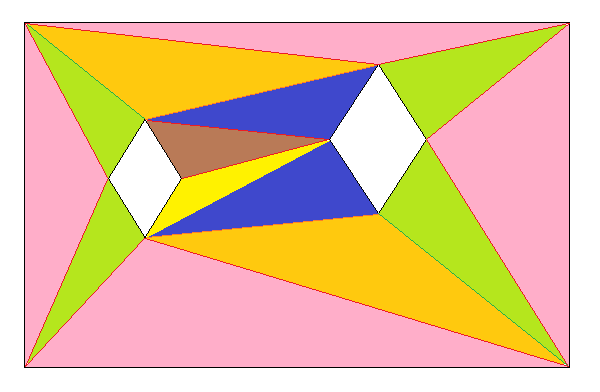# Is there a way to sort a list/array by vectors (instead of individual values)?

I have a plane with many vertices and two empty diamond holes:

Each intersection of the red lines and the 4 corners is a vertex, and that´s basically how it is supposed to look after procedurally ordering the triangles. I already have the vertex positions; but am struggling to find the triangle order, since not every row has the same amount of columns and viceversa. I HAVE AN IDEA, however, but it implies already having all of these vertices in order. I need them to be from left to right, from bottom to top.

The Sort() method ensures that they go in order, but as far as I can tell, it´s only with numbers (1, 2, 3, 4, 5…). I need the same, but with vectors. For example, (0, 1), (1, 1), (2, 1), (3, 1), (then it becomes a different row): (1, 2), (4, 2), (5, 2), (10, 2). where the number of rows and colums isn´t consistent, but prioritizes smaller z values and then, with rows with same z values, prioritizes smaller x values.
Does something like this exist?

I’m not sure I understand your conditions of sorting. Keep in mind that a sorting condition has to make sense for any two vertices you compare. So how would you actually decide which point should come before which? Of course you can simply sort them based on the y position first and if they happens to have the same y coordinate, sort them on the x coordinate. This can be done like this:

``````list.Sort((a, b) => { if (a.y == b.y) return a.x.CompareTo(b.x); return a.y.CompareTo(b.y); });
``````

``````list.Sort((a, b) => {
if (a.y == b.y) // if same y, sort on x
return a.x.CompareTo(b.x);
return a.y.CompareTo(b.y); // else sort on y
});
``````

So this will essentially give you all x coordinates sorted from lowest to largest if they are at the same y coordinate. Though the rows are essentially sorted bottom to top on the y axis.

Do you always have two diamond shaped holes? If so it would probably be cheaper to start with those edges and simply construct triangles connecting with the 4 corners. For exampleThe trick is to keep track of “unpaired” edges. Note that the edges need to be “facing” the right way. Unity’s mesh triangles follow a clockwise winding, so a hole would have a counter clockwise winding. The outer edges Would be clockwise, but need to be treated special since they don’t have any matching edge. Though you can simply create the first 4 triangles by picking an outer edge and create a triangle with the vertex that is closest. This will give you the 4 light red triangles. Of course whenever you create a triangle with an existing edge and a vertex you get two new edges. The edge you just used is now successfully paired and can be removed from the open edge list. So all you have to do is for each edge in the open list, just find the closest vertex in the “normal” direction of that edge. The normal of an edge would be the edge direction rotated clockwise 90°. So when you have the direction of the edge, you can get the normal by doing

``````Vector2 dir = end - start;
Vector2 normal = new Vector2(dir.y, -dir.x);
``````

With the normal you can simply measure the projection of all vertices onto your edge normal. Of course those “behind” the edge would have negative values which we will ignore. From the vertices on the positive side you simply grab the one with the smallest distance to the center of the edge. With that vertex and our edge we simply construct another triangle. Of course again the new triangle will have two new edges (of course oriented the right way).

Note before you push new edges into the open edge list, you should search the list if the counter part edge is already in the list. If it is, instead of adding the edge we have to remove the counter part since the edge is now already paired. This would happen for example when constructing the top left green triangle from the top left diamond edge. Since the left edge meet with the red triangle on the left, those edges are paired and done. Constructing the bottom left green triangle would actually remove two open edges from the two adjacent red triangles.
Continuing like this should fill the whole rectangle, leaving the holes free.

This should be just a matter of proper bookkeeping of the edges. Proper meshing, especially with holes, is not a simple topic. My solution should produce 14 triangles while your approach (which I think I don’t really understand) would result in 34 triangles (17 quads with 2 triangles each).

I should note that my approach is just a theoretical solution which I haven’t tried yet. It’s just based on what you can do easily. It should in theory work even with more holes as long as the hole shapes are “convex”.